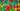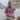# How to Use forEach() to Iterate an Array in JavaScript

Updated June 19, 2020Start discussion

What’s the usual thing you do with an array? Iterate through its items! This is where `forEach()` array method can be helpful.

This post describes how to use `forEach()` array method to iterate items of an array in JavaScript. Plus, you will read about `forEach()` best practices like correct handling of `this` and how to iterate array-like objects.

## 1. Basic forEach example

`array.forEach()` method iterates over the array items, in ascending order, without mutating the array.

The first argument of `forEach()` is the callback function called for every item in the array. The second argument (optional) is the value of `this` set in the callback.

``array.forEach(callback [, thisArgument])``

Let’s see how `forEach()` works in practice.

In the following example, `colors` array has 3 items. Let’s use `forEach()` to log to console every color:

``````const colors = ['blue', 'green', 'white'];

function iterate(item) {
console.log(item);
}

colors.forEach(iterate);
// logs "blue"
// logs "green"
// logs "white"``````

`iterate` a the callback function. `colors.forEach(iterate)` executes `iterate` function for every item in `colors`, setting the iterated item as the first argument.

This way, 3 invocation of `iterate()` function are perfomed:

• `iterate('blue')`
• `iterate('green')`
• `iterate('white')`

That’s how, in a few words, `forEach()` method works.

## 2. Index of the iterated element

`array.forEach(callback)` executes the `callback` function with 3 arguments: the current iterated item, the index of the iterated item and the array instance itself.

``array.forEach(callback(item [, index [, array]]))``

Let’s access the index of each item in the colors array:

``````const colors = ['blue', 'green', 'white'];

function iterate(item, index) {
console.log(`\${item} has index \${index}`);
}

colors.forEach(iterate);
// logs "blue has index 0"
// logs "green has index 1"
// logs "white has index 2"``````

`iterate()` function has access to the current iterated item and the index. The callback is executed 3 times:

• `iterate('blue', 0)`
• `iterate('green', 1)`
• `iterate('white', 2)`

## 3. Access the array inside the callback

To access the array itself during the iteration, you can use the 3rd parameter inside the callback function.

Let’s log the message `The last iteration!` when JavaScript executes the last iteration on the array items.

``````const colors = ['blue', 'green', 'white'];

function iterate(item, index, array) {
console.log(item);
if (index === array.length - 1) {
console.log('The last iteration!');
}
}

colors.forEach(iterate);
// logs "blue"
// logs "green"
// logs "white"
// logs "The last iteration!"``````

The 3rd parameter `array` inside the callback function is the array on which `forEach()` method was called on.

## 4. this inside the callback

Let’s run the following example in a browser, and pay attention to the value of `this`:

``````const letters = ['a', 'b', 'c'];

function iterate(letter) {
console.log(this === window); // true}

letters.forEach(iterate); // logs 3 times "true"``````

`this` inside `iterate()` equals to `window`, which is the global object in the browser environment. Follow regular function invocation to get more information.

In some situations, you might need to set `this` to an object of interest. Then indicate this object as the second argument when calling `forEach()`:

``array.forEach(callback, thisArgument)``

Let’s implement a `Unique` class, which always holds an unique list of items:

``````class Unique {
constructor(items) {
this.items = items;
}

append(newItems) {
newItems.forEach(function(newItem) {
if (!this.items.includes(newItem)) {
this.items.push(newItem);
}
}, this);      }
}

const uniqueColors = new Unique(['blue']);
console.log(uniqueColors.items); // => ['blue']

uniqueColors.append(['red', 'blue']);
console.log(uniqueColors.items); // => ['blue', 'red']``````

`newItems.forEach(function() {}, this)` is called with the second argument pointing to `this`, i.e. the instance of `Unique` class.

Inside the callback of `forEach()`, `this` points also to an instance of `Unique`. Now it’s safe to access `this.items`.

Note that for the above example using an arrow function as the callback of `forEach()` would be better. The arrow function preserves the value of `this` from the lexical scope, so there’s no need to use the second argument on `forEach()`.

## 5. forEach skips empty slots

`forEach()` skips the empty slots of the array (named sparse array).

``````const sparseArray = [1, , 3];
sparseArray.length; // => 3

sparseArray.forEach(function(item) {
console.log(item);
}); // logs 1, 3``````

`sparseArray` contains `1`, an empty slot, and `3`. `forEach()` iterates over `1` and `3`, but skips the empty slot.

## 6. Iterate array-like objects using forEach

`forEach()` can iterate over array-like objects:

``````const arrayLikeColors = {
"0": "blue",
"1": "green",
"2": "white",
"length": 3
};

function iterate(item) {
console.log(item);
}

Array.prototype.forEach.call(arrayLikeColors, iterate);// logs "blue"
// logs "green"
// logs "white"``````

`arrayLikeColors` is an array-like object. In order to iterate over its items, you have to call indirectly `forEach()` using the `call()`. The `forEach()` method is taken from `Array.prototype`.

Alternatively, you can transform the array-like object into an array using `Array.from()`, then iterate:

``````const arrayLikeColors = {
"0": "blue",
"1": "green",
"2": "white",
"length": 3
};

function iterate(item) {
console.log(item);
}

Array.from(arrayLikeColors).forEach(iterate);// logs "blue"
// logs "green"
// logs "white"``````

## 7. When to use forEach()

`forEach()` is best used to iterate array items, without breaking, and having simultaneously some side-effect.

Side-effects examples are a mutation of an outer scope variable, I/O operations (HTTP requests), DOM manipulations, and alike.

For example, let’s select all input elements from the DOM and use `forEach()` to clear them:

``````const inputs = document.querySelectorAll('input[type="text"]');

inputs.forEach(function(input) {
input.value = '';
});``````

The side effect in the callback function is clearing the value of the input field.

Keep in mind that you cannot normally break the iteration of `forEach()` (other than a tricky way to throw an error to stop the iteration, which is a cheap hack). The method will always iterate over all the items.

If your case requires an early break from the cycle, a better option is the classic for or for..of.

When the array iteration computes a result, without side-effects, a better alternative is to select an array method like:

For example, let’s determine whether all numbers of an array are even.

The first solution involves `forEach()` method:

``````let allEven = true;

const numbers = [22, 3, 4, 10];

numbers.forEach(function(number) {
if (number % 2 === 1) {
allEven = false;
// Break here
}
});

console.log(allEven); // => false``````

The code determines correctly if all numbers are even. The problem is the impossibility to break after finding the first odd number `3`.

For this situation, a better alternative is `array.every()` method:

``````const numbers = [22, 3, 4, 10];

const allEven = numbers.every(function(number) {
return number % 2 === 0;
});

console.log(allEven); // => false``````

`array.every()` doesn’t only make the code shorter. It is also optimal, because `.every()` method breaks iterating after finding the first odd number.

## 8. Conclusion

`array.forEach(callback)` method is an efficient way to iterate over all array items. Its first argument is the `callback` function, which is invoked for every item in the array with 3 arguments: item, index, and the array itself.

`forEach()` is useful to iterate over all array items, without breaking, involving simultaneously some side-effects. Otherwise, consider an alternative array method.

Do you know good uses cases of `forEach()`? Write them in a comment below.

## Quality posts into your inbox

I regularly publish posts containing:

• Important JavaScript concepts explained in simple words
• Overview of new JavaScript features
• How to use TypeScript and typing
• Software design and good coding practices